# The growth in the number​ (in millions) of Internet users in a certain country between 1990 and 2015 can be approximated by a logistic function withk=0.0016where t is the number of years since 1990. In 1990​ (whent=​0),the number of users was about44​million, and the number is expected to level out around220million.​(a) Find the growth function​ G(t) for the number of Internet users in the country.Estimate the number of Internet users in the country and the rate of growth for the following years.​(b) 1994​(c) 2001​(d) 2010 ​(e) What happens to the rate of growth over​ time?​(a)​ G(t)equals=(INSERT ANSWER HERE)

Question
19 views
The growth in the number​ (in millions) of Internet users in a certain country between 1990 and 2015 can be approximated by a logistic function with
k=0.0016
where t is the number of years since 1990. In 1990​ (when
t=​0),
the number of users was about
44
​million, and the number is expected to level out around
220
million.
 ​(a) Find the growth function​ G(t) for the number of Internet users in the country. Estimate the number of Internet users in the country and the rate of growth for the following years. ​(b) 1994 ​(c) 2001 ​(d) 2010 ​(e) What happens to the rate of growth over​ time?
​(a)
check_circle

Step 1

The logistic function is given as,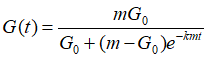,

where G0=initial number

m= maximum possible number

t=time

k=constant

Step 2

(a) In the given problem,

G0=44 million

m  = 220 million

k= 0.0016

plugging all these vlaues in the logistic function,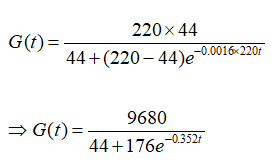(b) in year 1994,

t=4 years, ( since stating year is 1990)

plugging t=4 in G(t),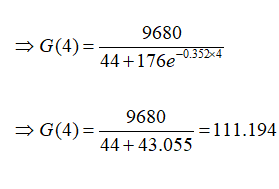(c) in year 2001,

t=11 years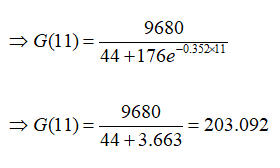(d) in year 2010,

t=20 years,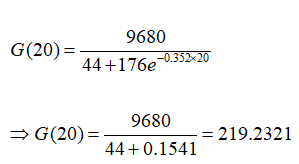(e) From the above cases, we see that as the year goes up, the growth rate increases.

...

### Want to see the full answer?

See Solution

#### Want to see this answer and more?

Solutions are written by subject experts who are available 24/7. Questions are typically answered within 1 hour.*

See Solution
*Response times may vary by subject and question.
Tagged in